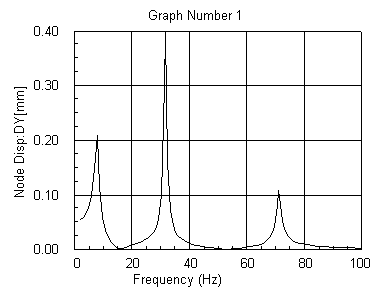# Strand7 harmonic response solver

Harmonic analysis is used to predict the steady state dynamic response of a structure subjected to sinusoidally varying loads. Strand7 allows two types of harmonic loading of which only one type may be included in a given solution.

All externally applied nodal, element, gravity and thermal loads are included in this type of load input. Any number of load cases may be used to define the loading condition and included in the solution. Load components in each load case use the same factor and phase angle. Different load cases may have different factors and phase angles, but the frequency for all loads is the same.

Base Excitation
The structure is excited harmonically at the fixed degrees of freedom. The excitation is defined by a direction vector of displacement, velocity or acceleration. This type of loading is particularly useful for modelling shaker table tests, as the base nodes are often the locations where nodal degrees of freedom are fixed.

The harmonic response solver is based on a mode superposition technique and performs the following steps:

• Calculates and assembles the element mass matrix if base excitation loading is applied, otherwise calculates and assembles the element load and nodal load vectors. The type of mass matrix used depends on the type used in the natural frequency analysis.

• Calculates modal loads and associated phase angles.

• Evaluates modal damping if Rayleigh damping is applied.

• Calculates modal element stresses and strains and nodal reactions using the vibration mode vectors.

• Calculates the modal displacement amplitudes and phase angles.

• Calculates the structure's responses such as nodal displacements, reactions and element stresses by combining the modal results. Both response magnitudes and phase angles are determined.

### Notes

1. The analysis relies on natural frequency results.

2. The same natural frequency result file can be used to run the harmonic solver as many times as needed with different harmonic solution settings such as frequency range and damping ratio values.

3. Theoretically, if all free vibration modes are included in the analysis, the results will be exact. Otherwise the solution will only be an approximate one. Generally, results that are more accurate can be expected when more modes are included. To achieve reasonably accurate results, all free vibration modes within and near the specified frequency range, should be considered.

4. The response of the full structure is calculated by superposition of the modal responses considering the magnitudes and phase angles of the modal responses. This is based on a closed form solution.

5. For each forcing frequency step, an envelope of the maximum values of the response is given in the result file. When damping is included, these maximum values may not occur simultaneously and for this reason, the results for the phase angles are given. Output data includes the maximum values of nodal reaction, displacement, velocity, acceleration and phase angle as well as node and element forces, moments and stress.

6. All the displacement and stress results are available for each frequency step. The results for a single node or element may also be graphed as a function of frequency1. The solution is calculated at equally spaced steps over the user specified frequency range. Additional steps are introduced automatically at the natural frequencies (resonance frequencies) within the specified range to capture peak responses.

For more information on harmonic response analysis, see Strand7 Webnotes - Linear / Dynamics.